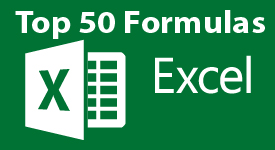Excel: Top 50 Microsoft Excel Formulas in 50 Minutes! | Bryan Hong | Skillshare

# Excel: Top 50 Microsoft Excel Formulas in 50 Minutes!

#### Bryan Hong, Online Teaching Excel Expert

Play Speed
• 0.5x
• 1x (Normal)
• 1.25x
• 1.5x
• 2x
22 Lessons (1h 3m)
• 1. Have Microsoft Excel Problems?

1:03
• 2. How to Get the Most from this Course

0:57
• 3. Text Formulas In Excel

7:53
• 4. Text Formulas Exercise

0:21
• 5. Text Formulas Exercise - Answers

1:08
• 6. Mathematical Formulas In Excel

4:01
• 7. Mathematical Formulas Exercise

0:16
• 8. Mathematical Formulas Exercise - Answers

1:00
• 9. Date and Time Formulas In Excel

5:04
• 10. Date and Time Formulas Exercise

0:17
• 11. Date and Time Formulas Exercise - Answers

0:59
• 12. Logic Formulas In Excel

12:20
• 13. Logic Formulas Exercise

0:15
• 14. Logic Formulas Exercise - Answers

0:41
• 15. Financial Formulas In Excel

5:54
• 16. Financial Formulas Exercise

0:13
• 17. Financial Formulas Exercise - Answers

2:15
• 18. Informational Formulas In Excel

3:58
• 19. Informational Formulas Exercise

0:40
• 20. Informational Formulas Exercise - Answers

1:26
• 21. Vlookup - Practical Examples

10:55
• 22. Thank You!

1:07
38 students are watching this class

Last time you opened a spreadsheet and are overwhelmed by the formulas and functions being used. You don't know how to make the most out of them, or change them into what you need.

But it doesn't have to be this way!

You Will Walk Away With...

• MORE TIME!
• Master the top 50 formulas in just 50 minutes!
• Understand the essence of these formulas, without memorizing them!
• See how each of these formulas is used with real examples!

After this class you will be able to:

• Actually SMILE when you open a spreadsheet :-)
• Brag to your friends about how you can use Excel formulas and Excel functions confidently!

If you're like me, you use Microsoft Excel on a daily basis for important tasks, text processing, or calculations. Whether it's for business or personal related projects, everyone wants to be able to use these formulas freely and easily.If you're ready to gain full control of Excel Formulas TODAY, take the “Excel -Master the Top 50 Formulas in 50 minutes from Scratch" course right now and master the following formulas:

• Text Formulas:
• Concatenate
• Find
• Left
• Len
• Lower
• Mid
• Proper
• Rept
• Substitute
• Trim
• Upper
• Value
• Mathematical Formulas:
• Average
• Int
• Mod
• Rand
• Round
• Sum
• Date and Time Formulas:
• Day
• Hour
• Minute
• Month
• Networkdays
• Now
• Second
• Today
• Weekday
• Year
• Logic Formulas:
• And
• Countblank
• Countif
• If
• Large
• Max
• Min
• Not
• Or
• Small
• Sumif
• Vlookup
• Financial Formulas:
• Fv
• Npv
• Pmt
• Ppmt
• Informational Formulas:
• Column
• Isblank
• Iserror
• Isnumber
• Istext
• Row